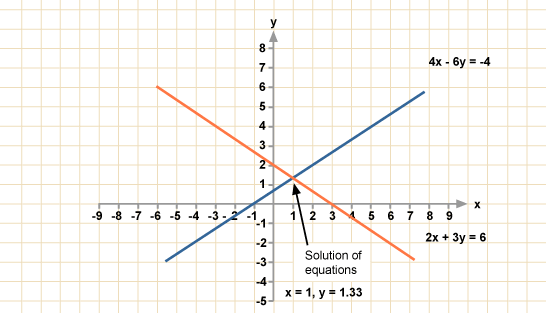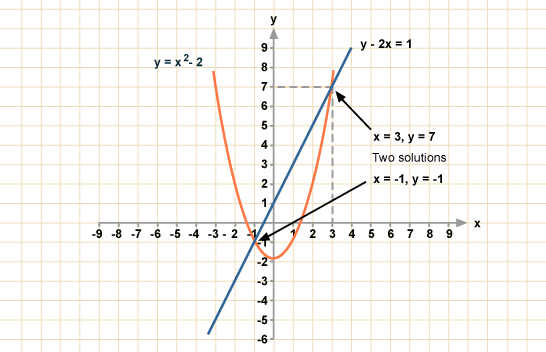Solving simultaneous equations using graphs - EDUSPORTSTZ

#Solving simultaneous equations using a graph is easier than you might think. First, you need to draw the lines of the equations. The points where the lines cross is the solution.

## Linear equations

The graphs of linear equations will give straight lines.

### Example

• Solve these simultaneous equations by drawing graphs:
• 2x + 3y = 6
• 4x - 6y = - 4
For example, to draw the line 2x + 3y = 6 pick two easy numbers to plot. One when x = 0 and one where y= 0
• When x = 0 in the equation 2x + 3y = 6
• This means 3y = 6 so y = 2
• So one point on the line is (0, 2)
• When y = 0
• 2x = 6 so x = 3
• So another point on the line is (3 ,0)
In an exam, only use this method if you are prompted to by a question. It is usually quicker to use algebra if you are not asked to use graphs.## Linear and quadratic equation

### Example

• Solve the simultaneous equations by drawing graphs.
• y - 2x = 1
• y = x2 - 2